Latest Banking jobs   »

# Quantitative Aptitude Quiz For RBI Assistant/ ESIC UDC Mains 2022- 13th April

Directions (1-10): In each of these questions, two equations (I) and (II) are given, you have to solve both the equations and give answer.

Q1. I. 20x²-41x+20=0
II. 2y²-19y+30=0
(a) if x < y
(b) if x > y
(c) if x ≤ y
(d) if x ≥ y
(e) if x = y, or relationship between x and y can’t be established.

Q2. I. 5x²+32x+51=0
II. 7y²-37y-440=0
(a) if x < y
(b) if x > y
(c) if x ≤ y
(d) if x ≥ y
(e) if x = y, or relationship between x and y can’t be established.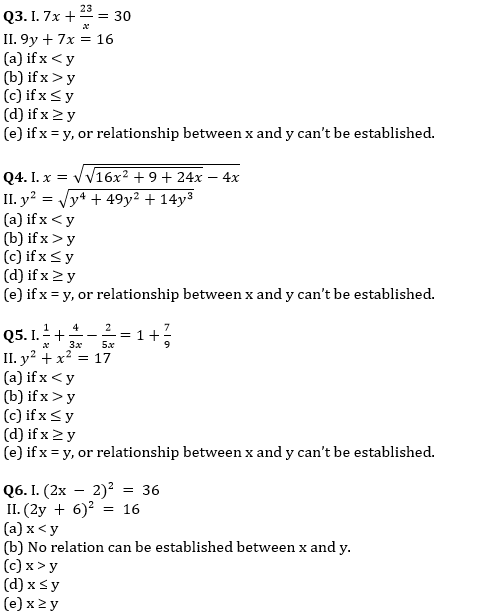Q7. I. 2x² – 29x + 90=0
II.2y² – 32y + 126 = 0
(a) x > y
(b) x ≤ y
(c) x ≥ y
(d) x < y
(e) No relation can be established between x and y.

Q8. I. x² + 156 – 25x = 0
II. y²- 5y + 6 = 0
(a) x ≤ y
(b) x < y
(c) x > y
(d) No relation can be established between x and y.
(e) x ≥ y

Q9. I. 4x² – 30x + 56 = 0
II. 5y² – 8y + 3 = 0
(a) x > y
(b) x ≤ y
(c) x ≥ y
(d) x < y
(e) No relation can be established between x and y.

Q10. I. 8x² + 17x – 21 = 0
II. y² + 21y + 90 = 0
(a) x ≤ y
(b) x < y
(c) x > y
(d) No relation can be established between x and y.
(e) x ≥ y

Solutions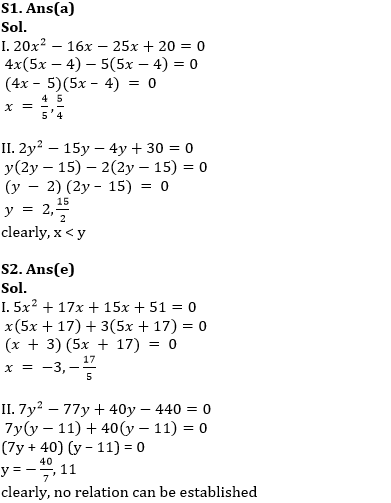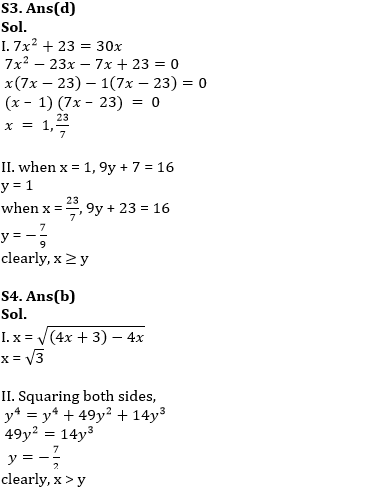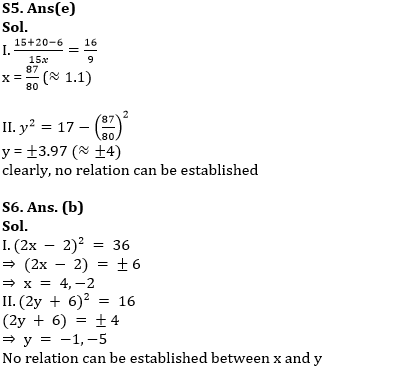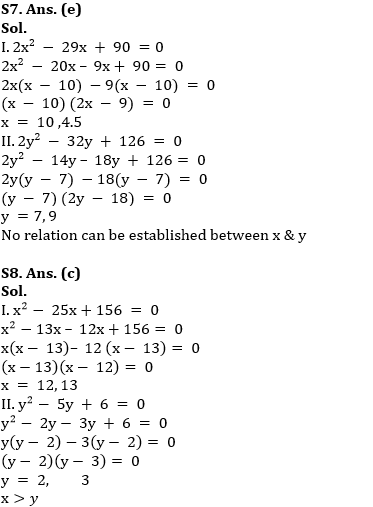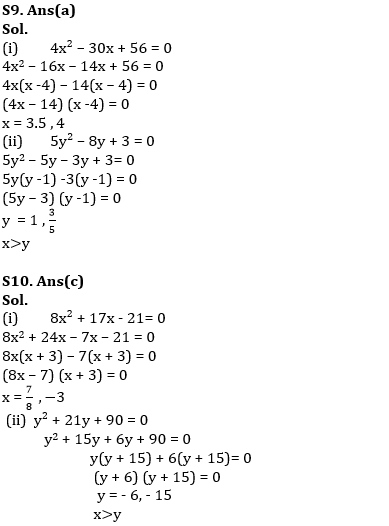#### Congratulations!Download Hindu Review of October 2021: Free PDF